# Page numbering

The book has 88 pages. How many times is the number 4 used for the book numbering?

n =  18

### Step-by-step explanation:Did you find an error or inaccuracy? Feel free to write us. Thank you!Tips to related online calculators
Would you like to compute count of combinations?

## Related math problems and questions:

• A bookA book contains 524 pages. If it is known that a person will select any one page between the pages numbered 125 and 384, find the probability of choosing the page numbered 252 or 253.
• 1 pageOne page is torn from the book. The sum of the page numbers of all the remaining pages is 15,000. What numbers did the pages have on the page that was torn from the book?
• Pages countingThere are pages numbered from 2 to 104 in the book. How many digits have to be printed to number the pages?
• Book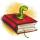Louise read from their favorite book for three days, 18 pages. Every day read the same number of pages. How many pages do she read every day?
• Every day 7 pagesAdelka reads the book 7 pages every day. When she reads one more page a day, she will read it three days earlier. How long will Adelka read a book? How much does a book of pages have?
• Book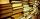Jitka read on holidays book that has 180 pages. In the first week read 45 pages. In the second week she read 15 pages more than the first week. How many pages left to read it yet?
• Book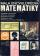To number pages of the thick book was used 4201 digits. How many pages has this book?
• Number 4Kamila wrote all natural numbers from 1 to 400 inclusive. How many times did she write the number 4?
• Last pageTwo consecutive sheets dropped out of the book. The sum of the numbers on the sides of the dropped sheets is 154. What is the number of the last page of the dropped sheets?
• Pages of book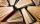Lenka calculated that if every day to read 16 pages of the book, read a book a day earlier than had read only 14 pages a day. How many pages has this book?
• MillieMillie has 3 sisters. Each sister reads 2 pages every morning and 4 pages every evening. Which expression can be used to represent the total number of pages read by Millie's sisters after 5 days?
• Borrowed book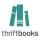Jane must as soon as possible return a borrowed book. She figured that when she read 15 pages a day return book in time. Then she read 18 pages a day and then return the book one day before. How many pages should have a book?
• Miguel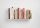Miguel and Kat are reading the same book. Miguel reads three times as many pages of the book as Kat. Together they read 48 pages. Write an equation that can be used to find how many pages Kat read.
• Book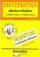Calculate the thickness of the page of the collection of examples from mathematics, where 4.87 cm thick and has 451 pages.
• Subtraction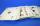How many times you can subtract the number 4 from the number 64?
• Claire and bookAfter Claire has read the first 5/8 of the book, there are 120 pages left to read. What is the total number of pages of the book?If we read the book at a speed of 15 pages a day, we read it 3 days before we read it at a speed of 10 pages per day. If I read at 6 pages per day, how many days will I read the book?